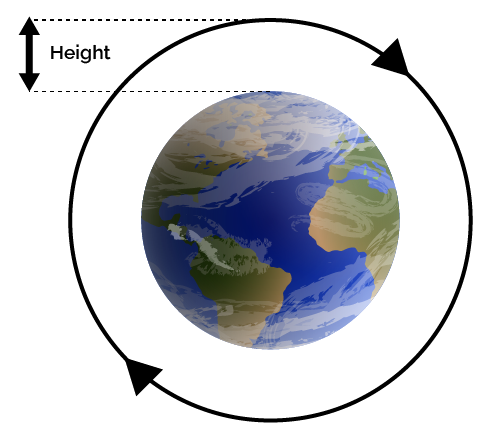# Earth Orbit Calculator

Created by Juhi Raj, PhD candidate
Reviewed by Steven Wooding
Last updated: Sep 29, 2022

This Earth orbit calculator helps you determine the orbital speed and orbital period of Earth's satellites at a given height above the average earth sea level. The fundamental laws that control the motion of Earth's satellites around Earth apply to the motion of planets, the moon, the earth's only natural satellite, and other satellites in the skies. Read on to learn about how different attributes of the satellite rotation are determined by its distance from the Earth's surface.

## What are earth's satellites?

A satellite is a small object that orbits another larger object. The earth is a satellite because it orbits the sun. We consider the moon a satellite because it orbits the earth. However, most people think of an artificial satellite when they say satellite. These spacecraft are launched into an orbit around the earth or any other celestial body. Sputnik 1 was Earth's first artificial satellite. It was launched into an elliptical low Earth orbit at the height of 939 km. It orbited for three weeks before its batteries ran out.

There are tens of thousands of artificial satellites orbiting the earth. Some photograph our globe, while others photograph other planets, the sun, and other celestial bodies. These images aid scientists in studying the earth, the solar system, and the universe. Other satellites broadcast television and make phone calls all around the world.

This Earth orbit calculator has two features to help you calculate the orbital speed and period of rotation of an Earth's satellite launched at a specific height from the surface of the earth.

## How to calculate the orbital speed of a satellite using this earth orbit calculator

According to Nicolaus Copernicus, earth and the other planets orbit the Sun in circles. He also discovered that as the distance from the Sun increased, so did the orbital periods. Kepler later found these orbits to be ellipses, but the orbits of most planets in the solar system are roughly circular. The Earth's orbital distance from the Sun fluctuates by only 2%. The eccentric orbit of Mercury, whose orbital distance changes by approximately 40%, is an exception.

Determining a satellite's orbital speed and period is significantly easier in circular orbits. We use this simplification in the following calculations. This tool focuses on objects orbiting the Earth, but you can apply our findings to other situations. Therefore, the orbital speed (see orbital velocity calculator) of an earth's satellite is given as follows:

$\small \text{orbital speed} = \sqrt{\frac{G \cdot M_{E}}{(R_{E}+h)}}$

where:

• $G$ – Earth's gravitational constant;
• $M_E$ – Earth's mass;
• $R_E$ – Earth's radius; and
• $h$ – Perpendicular distance of the satellite from the surface of the earth.

You can simply calculate this using this Earth orbit calculator by selecting the option Speed of the satellite, entering the height of the object rotating the earth, and voila, you have the orbital speed of the earth's satellite!

You need a certain amount of speed to reach the orbit (and stay there): learn how to calculate it with our delta-$v$ calculator!

## How can you estimate the period of rotation for an earth's satellite?

Similar to the earth's orbit, all of the earth's satellites orbit the earth at a certain height with a constant period of rotation.

Therefore, the orbital period of an earth's satellite is given as follows:

$\small \text{orbital period} = 2\pi \sqrt{\frac{(R_{E}+h)^3}{G \cdot M_{E}}}$

Orbital period
Find out more on how to calculate orbital period using our dedicated tool on the subject.

You can select the option Period of satellite rotation; enter the height of the object rotating the earth; you now know the orbital period of the earth's satellite!

## Example on how to use this earth orbit calculator

Let's try to determine the orbital speed and period for the International Space Station (ISS)

Since the ISS orbits at a height of 400 km above Earth's surface, the radius at which it orbits is RE + 400 km.

You can just plug in the height of the ISS from the earth's surface as 400 km, and the calculator will compute its orbital period as 1.54 hrs and orbital speed as 7.672 km/s.

## FAQ

### How do you calculate the orbital speed of an earth's satellite?

To calculate the orbital speed of an earth's satellite, you need to know the gravitational constant (G), earth's mass (M), earth's radius (R), and the height of rotation of the satellite (h).

The orbital speed is calculated as:

• √((G × M) / (R + h))

### What determines the orbital period of a satellite?

The orbital period of a satellite depends on the mass of the planet being orbited and the distance of the satellite from the center of the planet.

We can obtain the orbital period of a satellite T from Newton's form of Kepler's third law.

• T2 = 4 π a3 / GM

where G is the gravitational constant and M is the mass of the earth.

Juhi Raj, PhD candidate
Select
Period of satellite rotation
Height
mi
Orbital period
hrsHeight of the satellite from the Earth.
People also viewed…

### Alien civilization

The alien civilization calculator explores the existence of extraterrestrial civilizations by comparing two models: the Drake equation and the Astrobiological Copernican Limits👽

### Energy to wavelength

Have the energy of a wave or photon, and want to find its wavelength? Look no further than the energy to wavelength calculator!

### Plant spacing

Planning out your garden? Try the plant spacing calculator.

### Relativistic kinetic energy

The relativistic kinetic energy calculator finds the kinetic energy of an object traveling at a speed similar to the speed of light.